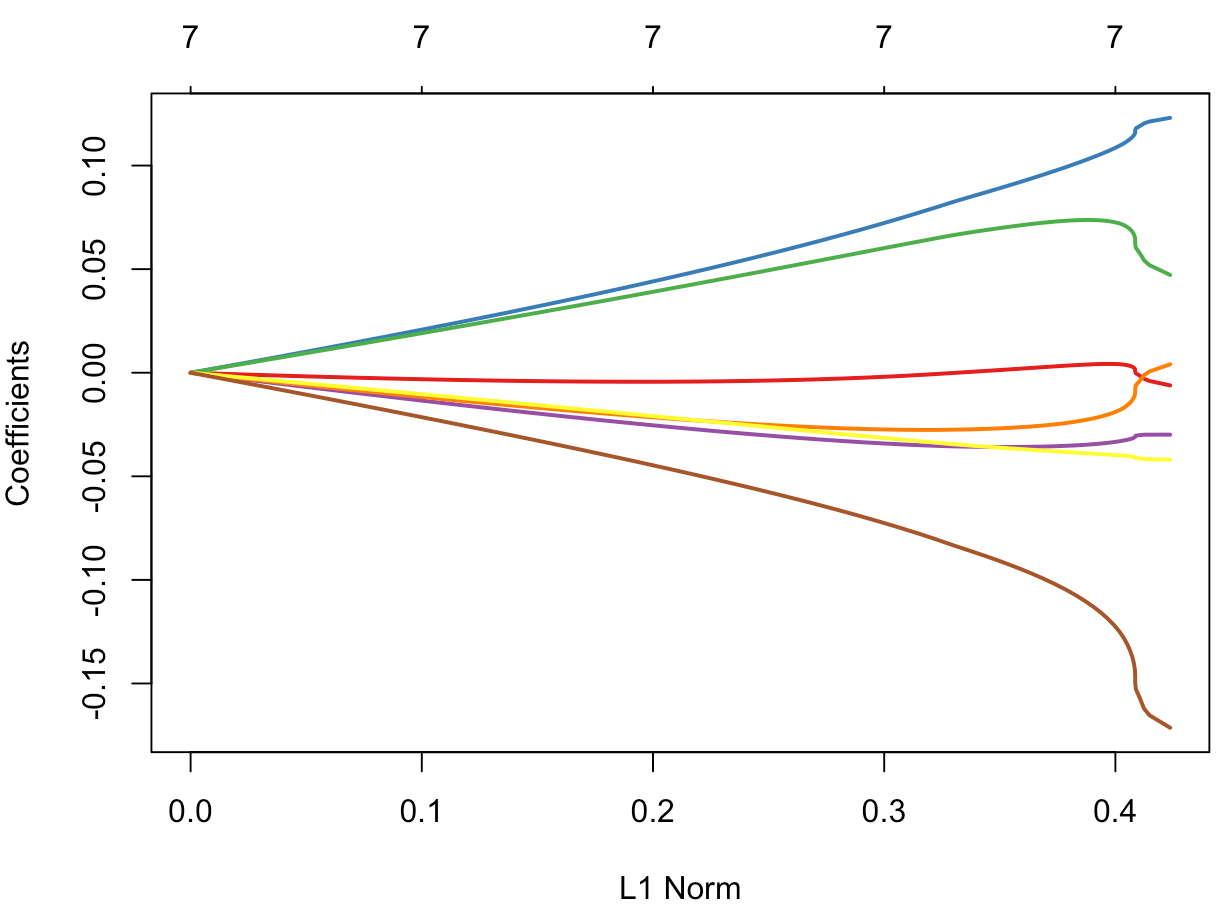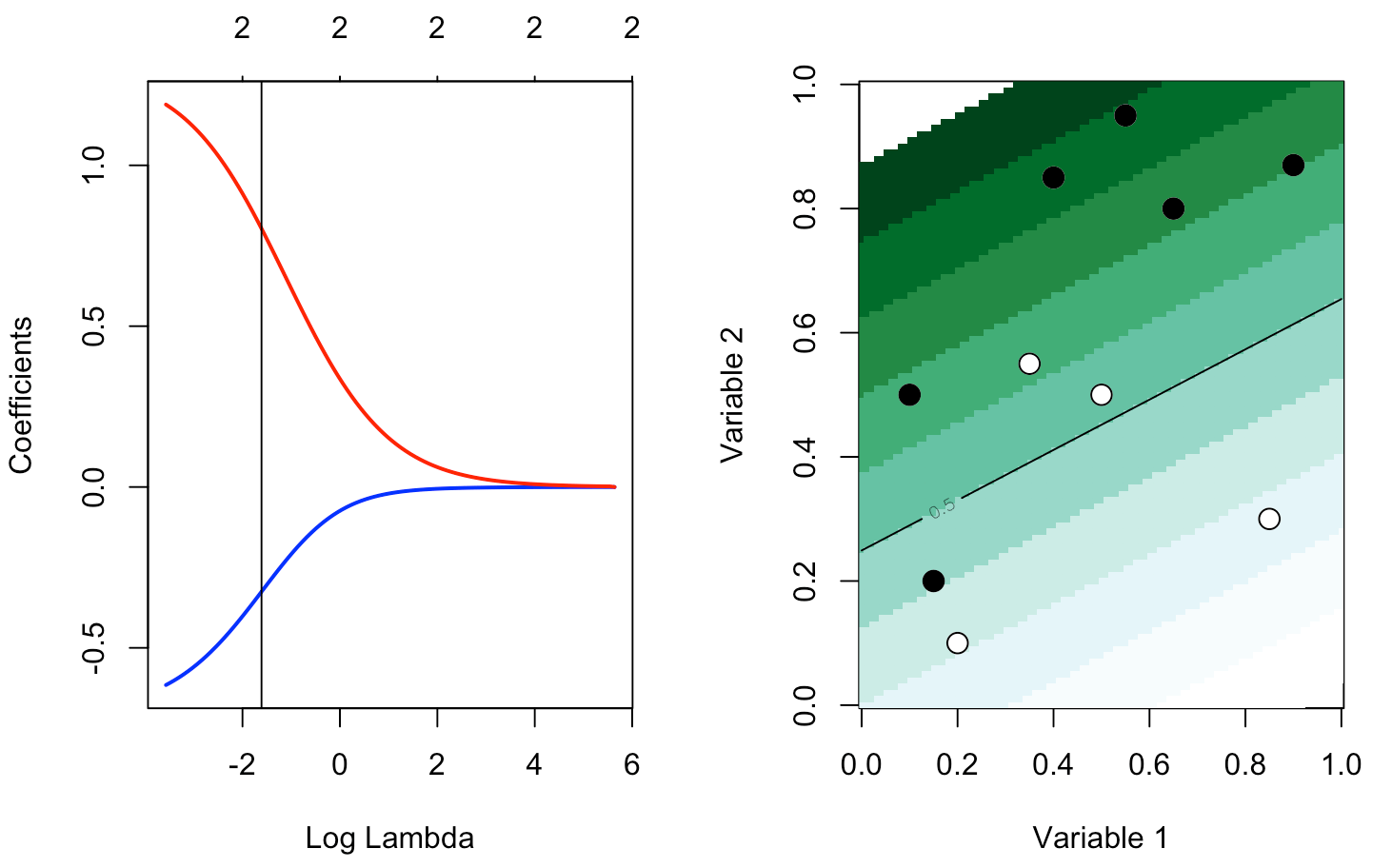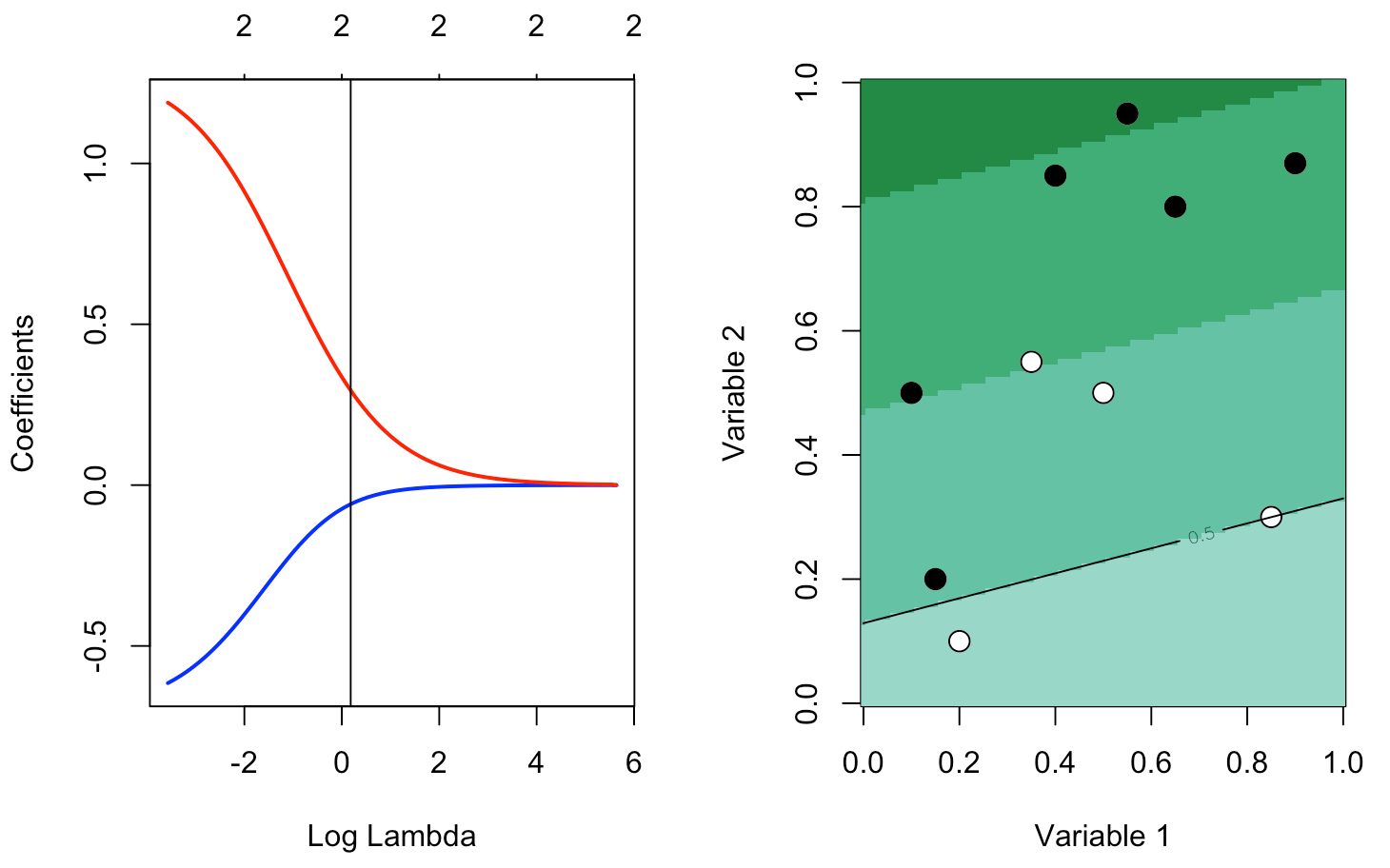Want to share your content on R-bloggers? click here if you have a blog, or here if you don't.

Fourth post of our series on classification from scratch, following the previous post which was some sort of detour on kernels. But today, we’ll get back on the logistic model.

## Formal approach of the problem

We’ve seen before that the classical estimation technique used to estimate the parameters of a parametric model was to use the maximum likelihood approach. More specifically, [latex display=”true”]\widehat{\mathbf{\beta}}=\text{argmax}\lbrace \log\mathcal{L}(\mathbf{\beta}|\mathbf{x},\mathbf{y})\rbrace[/latex]The objective function here focuses (only) on the goodness of fit. But usually, in econometrics, we believe something like non sunt multiplicanda entia sine necessitate (“entities are not to be multiplied without necessity”), the parsimony principle, simpler theories are preferable to more complex ones. So we want to penalize for too complex models.

This is not a bad idea. It is mentioned here and there in econometrics textbooks, but usually, for model choice, not about the inference. Usually, we estimate parameters using maximum likelihood techniques, and them we use AIC or BIC to compare two models. Recall that Akaike (AIC) criteria is based on[latex display=”true”]-2\log\mathcal{L}(\widehat{\mathbf{\beta}}|\mathbf{x},\mathbf{y})+2\text{dim}(\widehat{\mathbf{\beta}})[/latex]We have on the left a measure for the goodness of fit, and on the right, a penalty increasing with the “complexity” of the model.

Very quickly, here, the complexity is the number of variates used. I will not enter into details about the concept of sparsity (and the true dimension of the problem), I will recommend to read the book by Martin Wainwright, Robert Tibshirani and Trevor Hastie on that issue. But assume that we do not make and variable selection, we consider the regression on all covariates. Define[latex display=”true”]\Vert\mathbf{a} \Vert_{\ell_0}=\sum_{i=1}^d \mathbf{1}(a_i\neq 0), ~~\Vert\mathbf{a} \Vert_{\ell_1}=\sum_{i=1}^d |a_i|,~~\Vert\mathbf{a} \Vert_{\ell_2}=\left(\sum_{i=1}^d a_i^2\right)^{1/2}[/latex]for any $\mathbf{a}\in\mathbb{R}^d$. One might say that the AIC could be written[latex display=”true”]-2\log\mathcal{L}(\widehat{\mathbf{\beta}}|\mathbf{x},\mathbf{y})+2\|\widehat{\mathbf{\beta}}\|_{\ell_0}[/latex]And actually, this will be our objective function. More specifically, we will consider
[latex display=”true”]\widehat{\mathbf{\beta}}_{\lambda}=\text{argmin}\lbrace -\log\mathcal{L}(\mathbf{\beta}|\mathbf{x},\mathbf{y})+\lambda\|\mathbf{\beta}\|\rbrace[/latex]for some norm $\|\cdot\|$. I will not get back here on the motivation and the (theoretical) properties of those estimates (that will actually be discussed in the Summer School in Barcelona, in July), but in this post, I want to discuss the numerical algorithm to solve such optimization problem, for $\|\cdot\|_{\ell_2}$ (the Ridge regression) and for $\|\cdot\|_{\ell_1}$ (the LASSO regression).

## Normalization of the covariates

The problem of $\|\mathbf{\beta}\|$ is that the norm should make sense, somehow. A small $\mathbf{\beta}_j$ is with respect to the “dimension” of $x_j$‘s. So, the first step will be to consider linear transformations of all covariates $x_j$ to get centered and scaled variables (with unit variance)

y = myocarde$PRONO X = myocarde[,1:7] for(j in 1:7) X[,j] = (X[,j]-mean(X[,j]))/sd(X[,j]) X = as.matrix(X) ## Ridge Regression (from scratch) Before running some codes, recall that we want to solve something like[latex display=”true”]\widehat{\mathbf{\beta}}_{\lambda}=\text{argmin}\lbrace -\log\mathcal{L}(\mathbf{\beta}|\mathbf{x},\mathbf{y})+\lambda\|\mathbf{\beta}\|_{\ell_2}^2\rbrace[/latex] In the case where we consider the log-likelihood of some Gaussian variable, we get the sum of the square of the residuals, and we can obtain an explicit solution. But not in the context of a logistic regression. The heuristics about Ridge regression is the following graph. In the background, we can visualize the (two-dimensional) log-likelihood of the logistic regression, and the blue circle is the constraint we have, if we rewite the optimization problem as a contrained optimization problem : [latex display=”true”]\min_{\mathbf{\beta}:\|\mathbf{\beta}\|^2_{\ell_2}\leq s} \lbrace \sum_{i=1}^n -\log\mathcal{L}(y_i,\beta_0+\mathbf{x}^T\mathbf{\beta}) \rbrace[/latex]can be written equivalently (it is a strictly convex problem)[latex display=”true”]\min_{\mathbf{\beta},\lambda} \lbrace -\sum_{i=1}^n \log\mathcal{L}(y_i,\beta_0+\mathbf{x}^T\mathbf{\beta}) +\lambda \|\mathbf{\beta}\|_{\ell_2}^2 \rbrace[/latex]Thus, the constrained maximum should lie in the blue disk LogLik = function(bbeta){ b0=bbeta beta=bbeta[-1] sum(-y*log(1 + exp(-(b0+X%*%beta))) - (1-y)*log(1 + exp(b0+X%*%beta)))} u = seq(-4,4,length=251) v = outer(u,u,function(x,y) LogLik(c(1,x,y))) image(u,u,v,col=rev(heat.colors(25))) contour(u,u,v,add=TRUE) u = seq(-1,1,length=251) lines(u,sqrt(1-u^2),type="l",lwd=2,col="blue") lines(u,-sqrt(1-u^2),type="l",lwd=2,col="blue")Let us consider the objective function, with the following code PennegLogLik = function(bbeta,lambda=0){ b0 = bbeta beta = bbeta[-1] -sum(-y*log(1 + exp(-(b0+X%*%beta))) - (1-y)* log(1 + exp(b0+X%*%beta)))+lambda*sum(beta^2) } Why not try a standard optimisation routine ? In the very first post on that series, we did mention that using optimization routines were not clever, since they were strongly relying on the starting point. But here, it is not the case lambda = 1 beta_init = lm(PRONO~.,data=myocarde)$coefficients
vpar = matrix(NA,1000,8)
for(i in 1:1000){
vpar[i,] = optim(par = beta_init*rnorm(8,1,2),
function(x) PennegLogLik(x,lambda), method = "BFGS", control = list(abstol=1e-9))$par} par(mfrow=c(1,2)) plot(density(vpar[,2]),ylab="",xlab=names(myocarde)) plot(density(vpar[,3]),ylab="",xlab=names(myocarde))Clearly, even if we change the starting point, it looks like we converge towards the same value. That could be considered as the optimum. The code to compute $\widehat{\mathbf{\beta}}_{\lambda}$ would then be opt_ridge = function(lambda){ beta_init = lm(PRONO~.,data=myocarde)$coefficients
logistic_opt = optim(par = beta_init*0, function(x) PennegLogLik(x,lambda),
method = "BFGS", control=list(abstol=1e-9))
logistic_opt$par[-1]} and we can visualize the evolution of $\widehat{\mathbf{\beta}}_{\lambda}$ as a function of ${\lambda}$ v_lambda = c(exp(seq(-2,5,length=61))) est_ridge = Vectorize(opt_ridge)(v_lambda) library("RColorBrewer") colrs = brewer.pal(7,"Set1") plot(v_lambda,est_ridge[1,],col=colrs) for(i in 2:7) lines(v_lambda,est_ridge[i,],col=colrs[i])At least it seems to make sense: we can observe the shrinkage as $\lambda$ increases (we’ll get back to that later on). ## Ridge, using Netwon Raphson algorithm We’ve seen that we can also use Newton Raphson to solve this problem. Without the penalty term, the algorithm was[latex display=”true”]\mathbf{\beta}_{new} = \mathbf{\beta}_{old} – \left(\frac{\partial^2\log\mathcal{L}(\mathbf{\beta}_{old})}{\partial\mathbf{\beta}\partial\mathbf{\beta}^T}\right)^{-1}\cdot \frac{\partial\log\mathcal{L}(\mathbf{\beta}_{old})}{\partial\mathbf{\beta}}[/latex]where [latex display=”true”]\frac{\partial\log\mathcal{L}(\mathbf{\beta}_{old})}{\partial\mathbf{\beta}}=\mathbf{X}^T(\mathbf{y}-\mathbf{p}_{old})[/latex]and[latex display=”true”]\frac{\partial^2\log\mathcal{L}(\mathbf{\beta}_{old})}{\partial\mathbf{\beta}\partial\mathbf{\beta}^T}=-\mathbf{X}^T\mathbf{\Delta}_{old}\mathbf{X}[/latex]where $\mathbf{\Delta}_{old}$ is the diagonal matrix with terms $\mathbf{p}_{old}(1-\mathbf{p}_{old})$ on the diagonal. Thus[latex display=”true”]\mathbf{\beta}_{new} = \mathbf{\beta}_{old} + (\mathbf{X}^T\mathbf{\Delta}_{old}\mathbf{X})^{-1}\mathbf{X}^T[\mathbf{y}-\mathbf{p}_{old}][/latex]that we can also write[latex display=”true”]\mathbf{\beta}_{new} =(\mathbf{X}^T\mathbf{\Delta}_{old}\mathbf{X})^{-1}\mathbf{X}^T\mathbf{\Delta}_{old}\mathbf{z}[/latex]where $\mathbf{z}=\mathbf{X}\mathbf{\beta}_{old}+\mathbf{\Delta}_{old}^{-1}[\mathbf{y}-\mathbf{p}_{old}]$. Here, on the penalized problem, we can easily prove that[latex display=”true”]\frac{\partial\log\mathcal{L}_p(\mathbf{\beta}_{\lambda,old})}{\partial\mathbf{\beta}}=\frac{\partial\log\mathcal{L}(\mathbf{\beta}_{\lambda,old})}{\partial\mathbf{\beta}}-2\lambda\mathbf{\beta}_{old}[/latex]while[latex display=”true”]\frac{\partial^2\log\mathcal{L}_p(\mathbf{\beta}_{\lambda,old})}{\partial\mathbf{\beta}\partial\mathbf{\beta}^T}=\frac{\partial^2\log\mathcal{L}(\mathbf{\beta}_{\lambda,old})}{\partial\mathbf{\beta}\partial\mathbf{\beta}^T}-2\lambda\mathbb{I}[/latex]Hence[latex display=”true”]\mathbf{\beta}_{\lambda,new} =(\mathbf{X}^T\mathbf{\Delta}_{old}\mathbf{X}+2\lambda\mathbb{I})^{-1}\mathbf{X}^T\mathbf{\Delta}_{old}\mathbf{z}[/latex] The code is then Y = myocarde$PRONO
X = myocarde[,1:7]
for(j in 1:7) X[,j] = (X[,j]-mean(X[,j]))/sd(X[,j])
X = as.matrix(X)
X = cbind(1,X)
colnames(X) = c("Inter",names(myocarde[,1:7]))
beta = as.matrix(lm(Y~0+X)$coefficients,ncol=1) for(s in 1:9){ pi = exp(X%*%beta[,s])/(1+exp(X%*%beta[,s])) Delta = matrix(0,nrow(X),nrow(X));diag(Delta)=(pi*(1-pi)) z = X%*%beta[,s] + solve(Delta)%*%(Y-pi) B = solve(t(X)%*%Delta%*%X+2*lambda*diag(ncol(X))) %*% (t(X)%*%Delta%*%z) beta = cbind(beta,B)} beta[,8:10] [,1] [,2] [,3] XInter 0.59619654 0.59619654 0.59619654 XFRCAR 0.09217848 0.09217848 0.09217848 XINCAR 0.77165707 0.77165707 0.77165707 XINSYS 0.69678521 0.69678521 0.69678521 XPRDIA -0.29575642 -0.29575642 -0.29575642 XPAPUL -0.23921101 -0.23921101 -0.23921101 XPVENT -0.33120792 -0.33120792 -0.33120792 XREPUL -0.84308972 -0.84308972 -0.84308972 Again, it seems that convergence is very fast. And interestingly, with that algorithm, we can also derive the variance of the estimator[latex display=”true”]\text{Var}[\widehat{\mathbf{\beta}}_{\lambda}]=[\mathbf{X}^T\mathbf{\Delta}\mathbf{X}+2\lambda\mathbb{I}]^{-1}\mathbf{X}^T\mathbf{\Delta}\text{Var}[\mathbf{z}]\mathbf{\Delta}\mathbf{X}[\mathbf{X}^T\mathbf{\Delta}\mathbf{X}+2\lambda\mathbb{I}]^{-1}[/latex]where$\text{Var}[\mathbf{z}]=\mathbf{\Delta}^{-1}$ The code to compute $\widehat{\mathbf{\beta}}_{\lambda}$ as a function of $\lambda$ is then newton_ridge = function(lambda=1){ beta = as.matrix(lm(Y~0+X)$coefficients,ncol=1)*runif(8)
for(s in 1:20){
pi = exp(X%*%beta[,s])/(1+exp(X%*%beta[,s]))
Delta = matrix(0,nrow(X),nrow(X));diag(Delta)=(pi*(1-pi))
z = X%*%beta[,s] + solve(Delta)%*%(Y-pi)
B = solve(t(X)%*%Delta%*%X+2*lambda*diag(ncol(X))) %*% (t(X)%*%Delta%*%z)
beta = cbind(beta,B)}
Varz = solve(Delta)
Varb = solve(t(X)%*%Delta%*%X+2*lambda*diag(ncol(X))) %*% t(X)%*% Delta %*% Varz %*%
Delta %*% X %*% solve(t(X)%*%Delta%*%X+2*lambda*diag(ncol(X)))
return(list(beta=beta[,ncol(beta)],sd=sqrt(diag(Varb))))}

We can visualize the evolution of $\widehat{\mathbf{\beta}}_{\lambda}$ (as a function of $\lambda$)

v_lambda=c(exp(seq(-2,5,length=61)))
est_ridge=Vectorize(function(x) newton_ridge(x)$beta)(v_lambda) library("RColorBrewer") colrs=brewer.pal(7,"Set1") plot(v_lambda,est_ridge[1,],col=colrs,type="l") for(i in 2:7) lines(v_lambda,est_ridge[i,],col=colrs[i])and to get the evolution of the variance v_lambda=c(exp(seq(-2,5,length=61))) est_ridge=Vectorize(function(x) newton_ridge(x)$sd)(v_lambda)
library("RColorBrewer")
colrs=brewer.pal(7,"Set1")
plot(v_lambda,est_ridge[1,],col=colrs,type="l")
for(i in 2:7) lines(v_lambda,est_ridge[i,],col=colrs[i],lwd=2)Recall that when $\lambda=0$ (on the left of the graphs), $\widehat{\mathbf{\beta}}_{0}=\widehat{\mathbf{\beta}}^{mco}$ (no penalty). Thus as $\lambda$ increase (i) the bias increase (estimates tend to 0) (ii) the variances deacrease.

## Ridge, using glmnet

As always, there are R functions availble to run a ridge regression. Let us use the glmnet function, with $\alpha=0$

y = myocarde$PRONO X = myocarde[,1:7] for(j in 1:7) X[,j] = (X[,j]-mean(X[,j]))/sd(X[,j]) X = as.matrix(X) library(glmnet) glm_ridge = glmnet(X, y, alpha=0) plot(glm_ridge,xvar="lambda",col=colrs,lwd=2)as a function of the normthe $\ell_1$ norm here, I don’t know why. I don’t know either why all graphs obtained with different optimisation routines are so different… Maybe that will be for another post… ## Ridge with orthogonal covariates An interesting case is obtained when covariates are orthogonal. This can be obtained using a PCA of the covariates. library(factoextra) pca = princomp(X) pca_X = get_pca_ind(pca)$coord

Let us run a ridge regression on those (orthogonal) covariates

library(glmnet)
glm_ridge = glmnet(pca_X, y, alpha=0)
plot(glm_ridge,xvar="lambda",col=colrs,lwd=2)plot(glm_ridge,col=colrs,lwd=2)We clearly observe the shrinkage of the parameters, in the sense that [latex display=”true”]\widehat{\mathbf{\beta}}_{\lambda}^{\perp}=\frac{\widehat{\mathbf{\beta}}^{mco}}{1+\lambda}[/latex]

## Application

Let us try with our second set of data

df0 = df
df0$y=as.numeric(df$y)-1
plot_lambda = function(lambda){
m = apply(df0,2,mean)
s = apply(df0,2,sd)
for(j in 1:2) df0[,j] = (df0[,j]-m[j])/s[j]
reg = glmnet(cbind(df0$x1,df0$x2), df0$y==1, alpha=0,lambda=lambda) u = seq(0,1,length=101) p = function(x,y){ xt = (x-m)/s yt = (y-m)/s predict(reg,newx=cbind(x1=xt,x2=yt),type='response')} v = outer(u,u,p) image(u,u,v,col=clr10,breaks=(0:10)/10) points(df$x1,df$x2,pch=c(1,19)[1+z],cex=1.5) contour(u,u,v,levels = .5,add=TRUE) } We can try various values of $\lambda$ reg = glmnet(cbind(df0$x1,df0$x2), df0$y==1, alpha=0)
par(mfrow=c(1,2))
plot(reg,xvar="lambda",col=c("blue","red"),lwd=2)
abline(v=log(.2))
plot_lambda(.2)or

reg = glmnet(cbind(df0$x1,df0$x2), df0\$y==1, alpha=0)
par(mfrow=c(1,2))
plot(reg,xvar="lambda",col=c("blue","red"),lwd=2)
abline(v=log(1.2))
plot_lambda(1.2)Next step is to change the norm of the penality, with the $\ell_1$ norm…